# Class 9 RD Sharma Solutions – Chapter 9 Triangles and its Angles- Exercise 9.2

• Last Updated : 08 Dec, 2020

### Question 1: The exterior angles, obtained on producing the base of a triangle both ways are 104° and 136°. Find all the angles of the triangle.

Solution: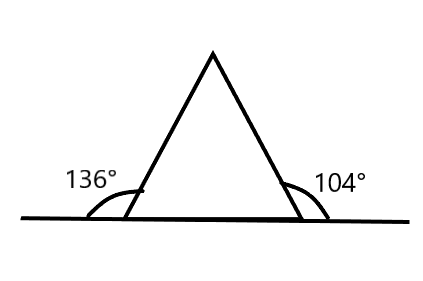Attention reader! All those who say programming isn't for kids, just haven't met the right mentors yet. Join the  Demo Class for First Step to Coding Coursespecifically designed for students of class 8 to 12.

The students will get to learn more about the world of programming in these free classes which will definitely help them in making a wise career choice in the future.

Theorems Used: The exterior angle theorem states that the measure of each exterior angle of a triangle is equal to the sum of the opposite and non-adjacent interior angles. (Exterior Angle Theorem)

∠ACD = ∠ABC + ∠BAC [Exterior Angle Theorem]

Find ∠ABC:

∠ABC + ∠ABE = 180° [Linear pair]

∠ABC + 136° = 180°

∠ABC = 44°

Find ∠ACB:

∠ACB + ∠ACD = 180° [Linear pair]

∠ACB + 1040 = 180°

∠ACB = 76°

Now,

Sum of all angles of a triangle = 180°

∠A + 44° + 76° = 180°

∠A = 180° − 44°−76°

∠ A = 60°

Angles of the triangle are ∠ A = 60°, ∠B = 44° and ∠C = 76° (ans)

### Question 2: In an △ABC, the internal bisectors of ∠B and ∠C meet at P and the external bisectors of ∠B and ∠C meet at Q. Prove that ∠BPC + ∠BQC = 180°.

Solution:

In △ABC,

BP and CP are an internal bisector of ∠B and ∠C respectively

=> External ∠B = 180° – ∠B

BQ and CQ are an external bisector of ∠B and ∠C respectively.

=> External ∠C = 180° – ∠C

In triangle BPC,

∠BPC + 1/2∠B + 1/2∠C = 180°

∠BPC = 180° – (∠B + ∠C) …. (1)

In triangle BQC,

∠BQC + 1/2(180° – ∠B) + 1/2(180° – ∠C) = 180°

∠BQC + 180° – (∠B + ∠C) = 180°

∠BPC + ∠BQC = 180° [Using (1)] (Proved)

### Question 3: In figure, the sides BC, CA and AB of a △ABC have been produced to D, E and F respectively. If ∠ACD = 105° and ∠EAF = 45°, find all the angles of the △ABC.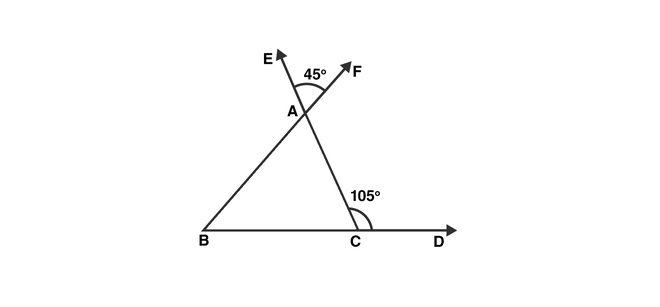Solution:

Theorems Used:

(i) The exterior angle theorem states that the measure of each exterior angle of a triangle is equal to the sum of the opposite and non-adjacent interior angles. (Exterior Angle Theorem)

(ii) Sum of a linear angle pair is 180°

(iii)Vertically opposite angles are equal.

∠BAC = ∠EAF = 45° [Vertically opposite angles]

∠ACD = 180° – 105° = 75° [Linear pair]

∠ABC = 105° – 45° = 60° [Exterior angle property]

### (i)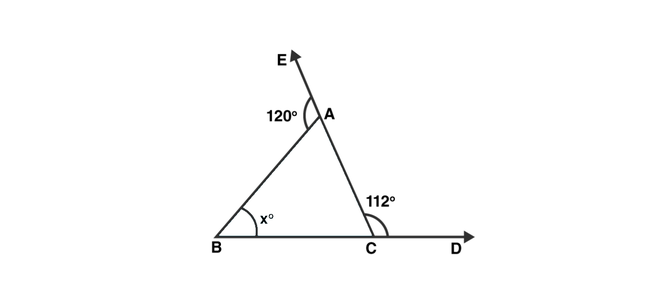Solution:

∠BAC = 180° – 120° = 60° [Linear pair]

∠ACB = 180° – 112° = 68° [Linear pair]

Sum of all angles of a triangle = 1800

x = 180° − ∠BAC − ∠ACB

= 180° − 60° − 68° = 52° (ans)

### (ii)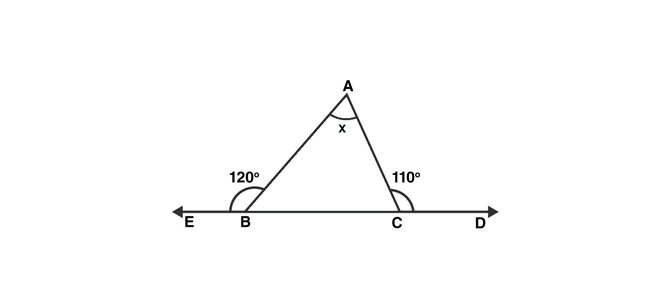Solution:

∠ABC = 180° – 120° = 60° [Linear pair]

∠ACB = 180° – 110° = 70° [Linear pair]

Sum of all angles of a triangle = 180°

x = ∠BAC = 180° − ∠ABC − ∠ACB

= 180° – 60° – 70° = 50° (ans)

### (iii)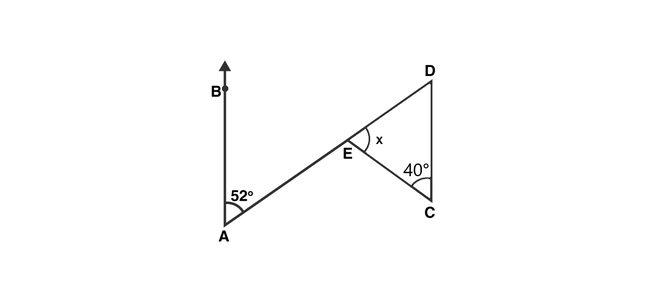Solution:

∠BAE = ∠EDC = 52° [Alternate angles]

Sum of all angles of a triangle = 180°

x = 180° – 40° – 52° = 180° − 92° = 88° (ans)

### (iv)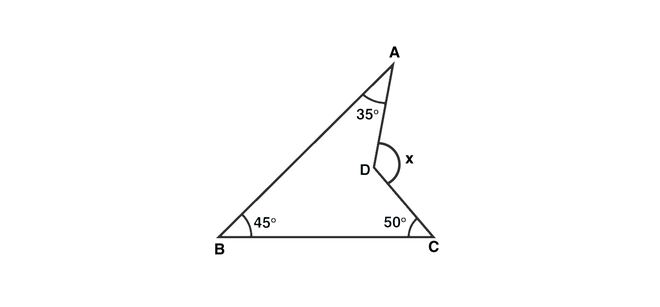Solution: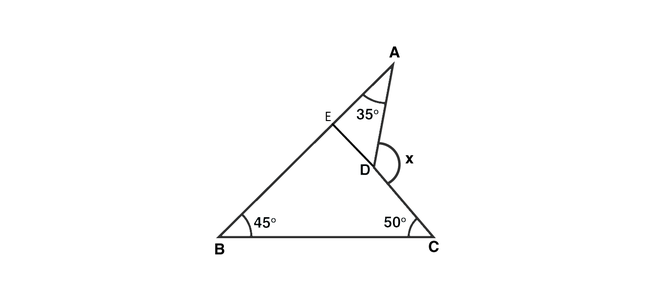CD is produced to meet AB at E.

∠BEC = 180° – 45° – 50° = 85° [Sum of all angles of a triangle = 180°]

∠AEC = 180° – 85° = 95° [Linear Pair]

Now, x = 95° + 35° = 130° [Exterior angle Property]

### Question 5: In thefigure, AB divides ∠DAC in the ratio 1 : 3 and AB = DB. Determine the value of x.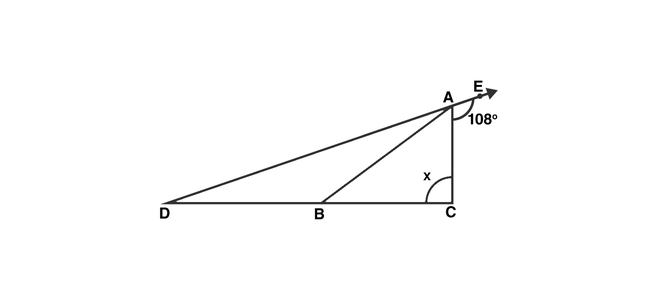Solution:

Let ∠BAD = y, ∠BAC = 3y

∠BDA = ∠BAD = y (As AB = DB)

Now,

∠BAD + ∠BAC + 108° = 180° [Linear Pair]

y + 3y + 108° = 180°

4y = 72°

or y = 18°

∠ADC + ∠ACD = 108° [Exterior Angle Property]

x + 18° = 180°

x = 90° (ans)

### Question 6: ABC is a triangle. The bisector of the exterior angle B and the bisector of ∠C intersect each other at D. Prove that ∠D = (1/2)∠A.

Solution: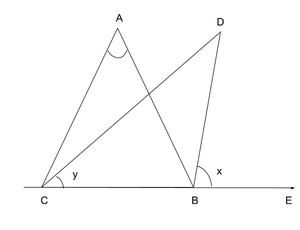Let ∠ABE = 2x and ∠ACB = 2y

∠ABC = 180° – 2K [Linear pair]

∠A = 180° — ∠ABC — ∠ACB [Angle sum property]

= 180° -180° + 2x – 2y

= 2(x – y)

Now, ∠D = 180° – ∠DBC – ∠DCB

∠D = 180° -(x + 180° – 2x) – y

= x – y

= (1/2)∠A (Hence Proved)

### Question 7: In the figure, AC is perpendicular to CE and ∠A:∠B:∠C =  3:2:1 Find ∠ECD.Solution:

Given that ∠A:∠B:∠C = 3:2:1

Let the angles be 3x, 2x and x.

3x + 2x + x = 180° [Angle Sum property]

6x = 180°

x = 30° = ∠ACB

Therefore,

∠ECD = 180° – ∠ACB – 90° [Linear Pair]

= 180° – 30° – 90°

= 60° (ans)

### Question 8: In the figure, AM is perpendicular to BC and AN is thebisector of ∠A. If ∠B = 65° and ∠C = 33° find ∠MAN.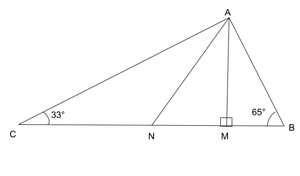Solution:

Let ∠BAN = ∠NAC = x [AN bisects ∠A]

Therefore, ∠ANM = x + 33° [Exterior angle property]

In △AMB,

∠BAM = 90° – 65° = 25° [Exterior angle property]

Therefore, ∠MAN = ∠BAN – ∠BAM = x – 25°

Now in △MAN,

(x – 25°) + (x + 33°) + 90° = 180° [Angle sum property]

or, 2x + 8° = 90°

or x = 41°

Therefore, ∠MAN = 41° – 25° = 16° (ans)

### Question 9: In a △ABC, AD bisects ∠A and ∠C > ∠B. Prove that ∠ADB > ∠ADC.

Solution: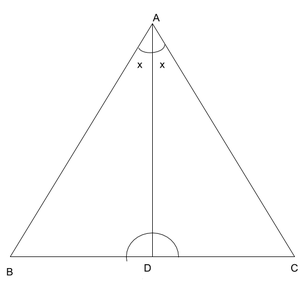Given that,

∠C > ∠B

or, ∠C + x > ∠B + x [Adding x on both sides]

### Question 10: In a △ABC, BD is perpendicular to AC and CE is perpendicular to AB. If BD and CE intersect at O prove that ∠BOC = 180° -∠A.

Solution: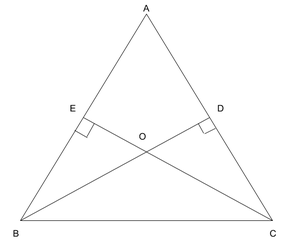∠A + ∠AEO + ∠EOD + ∠ADO = 360°

or, ∠A + 90° + 90° + ∠EOD = 360°

or, ∠A + ∠BOC = 360° – 90° – 90° [∠EOD = ∠BOC as they are vertically opposite angles]

or, ∠BOC = 180° – ∠A (proved)

### Question 11: In the figure, AE bisects ∠CAD and ∠B = ∠C. Prove that AE || BC.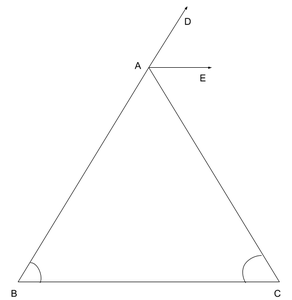Solution:

Let ∠B = ∠C = x

Then,

∠CAD = ∠B + ∠C = 2x (Exterior Angle)

∠EAC = ∠C [AE bisects ∠CAD and ∠C = x assumed]

These are interior angles for line AE and BC,

Therefore,

AE || BC (proved)

### Question 12: In the figure, AB || DE. Find ∠ACD.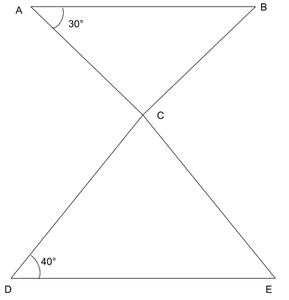Solution:

Since, AB || DE

Therefore,

∠ABC = ∠CDE = 40° [Alternate Angles]

∠ACB = 180° – ∠ABC – ∠BAC

= 180° – 40° – 30°

= 110°

Therefore,

∠ACD = 180° – ∠ACB [Linear Pair]

=70°

### Question 13. Which of the following statements are true (T) and which are false (F) :

(i) Sum of the three angles of a triangle is 180°.

(ii) A triangle can have two right angles.

(iii) All the angles of a triangle can be less than 60°.

(iv) All the angles of a triangle can be greater than 60°.

(v) All the angles of a triangle can be equal to 60°.

(vi) A triangle can have two obtuse angles.

(vii) A triangle can have at most one obtuse angles.

(viii) If one angle of a triangle is obtuse, then it cannot be a right-angled triangle.

(lx) An exterior angle of a triangle is less than either of its interior opposite angles.

(x) An exterior angle of a triangle is equal to the sum of the two interior opposite angles.

(xi) An exterior angle of a triangle is greater than the opposite interior angles.

### Question 14: Fill in the blanks to make the following statements true

(i) Sum of the angles of a triangle is _________.

(ii) An exterior angle of a triangle is equal to the two ____________ opposite angles.

(iii) An exterior angle of a triangle is always _________________ than either of the interior opposite angles.